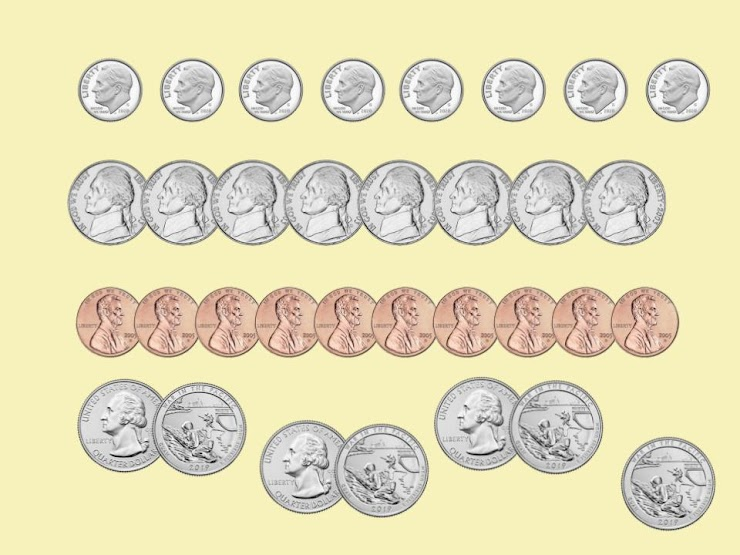Quarters 2
(Set 2) Identify and know the value of coins, and show different combinations of coins that equal the same value. Students will see the cent sign ¢ and the dollar sign \$ in this activity. Quarters, dimes, nickels & pennies only appear in this set, and the complexity of the coins being counted continues to increase.
From Mr. Anker Tests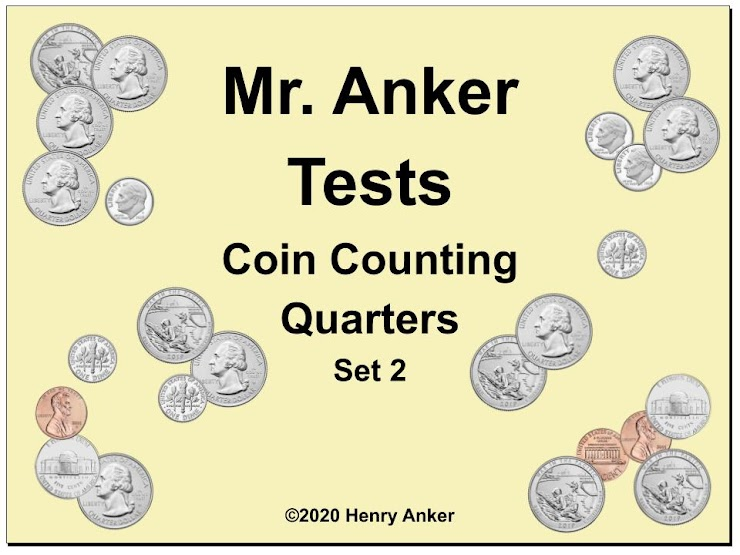Quarters Help Slide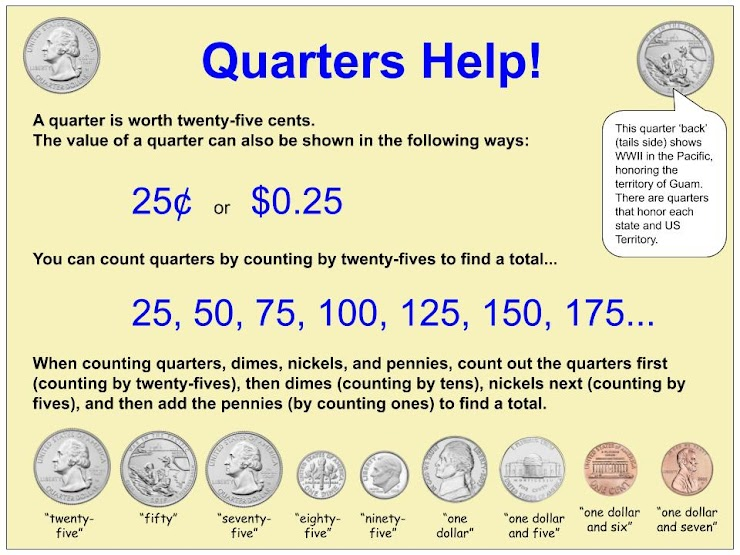1. What is the total value of the coins shown below?
1 point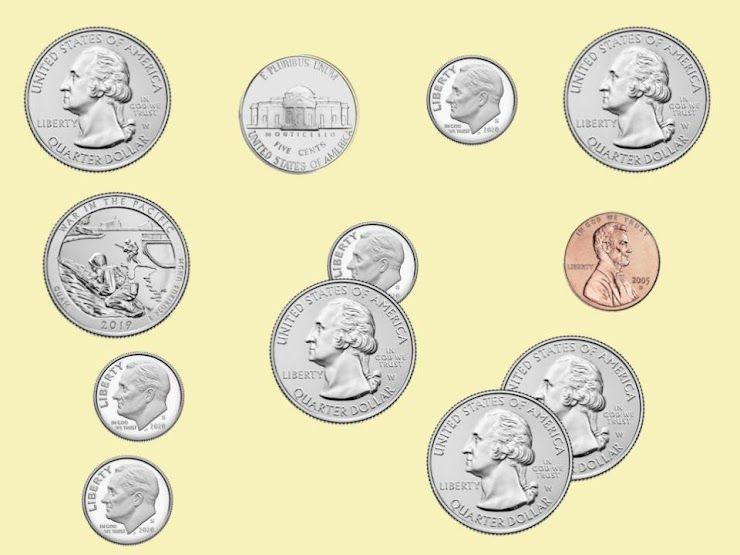2. Which set of coins shown below has the greatest value?
1 point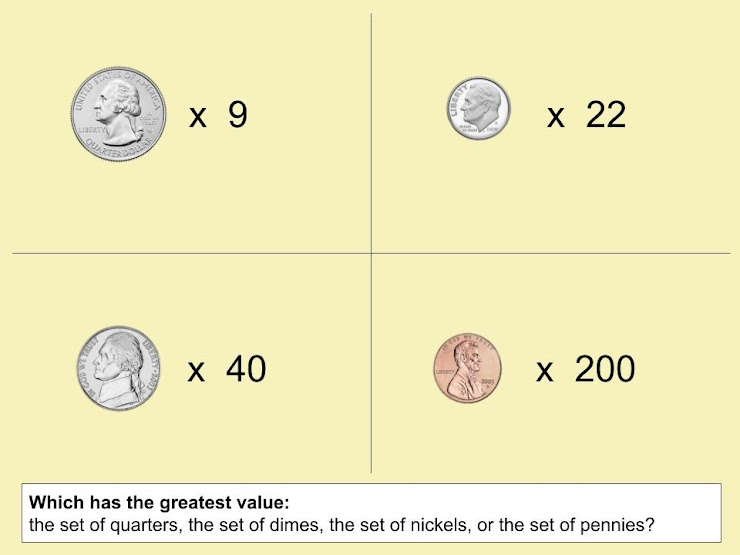3. What is the total value of the coins shown below?
1 point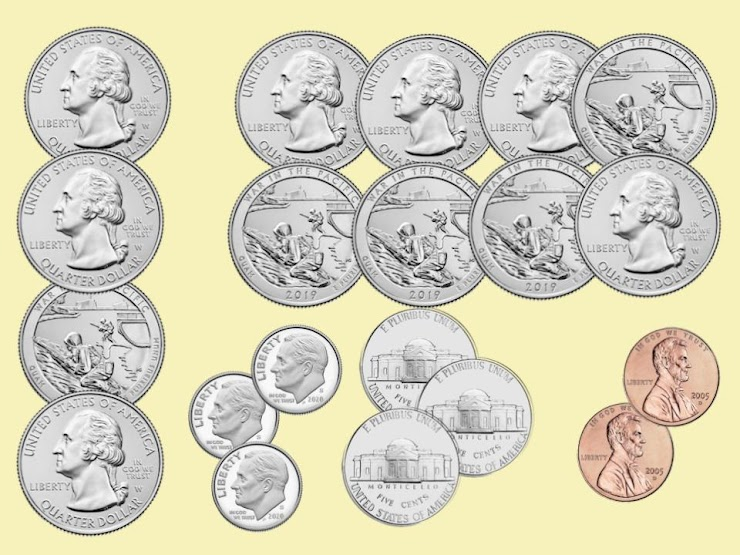4. Which of the options below has the least value?
1 point
5. Which set of coins shown below has the greatest value?
1 point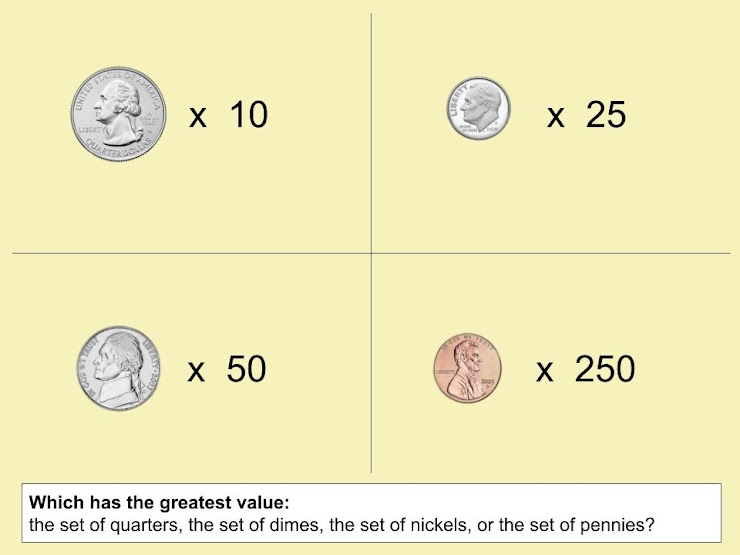6. What is the total value of the coins described here?
1 point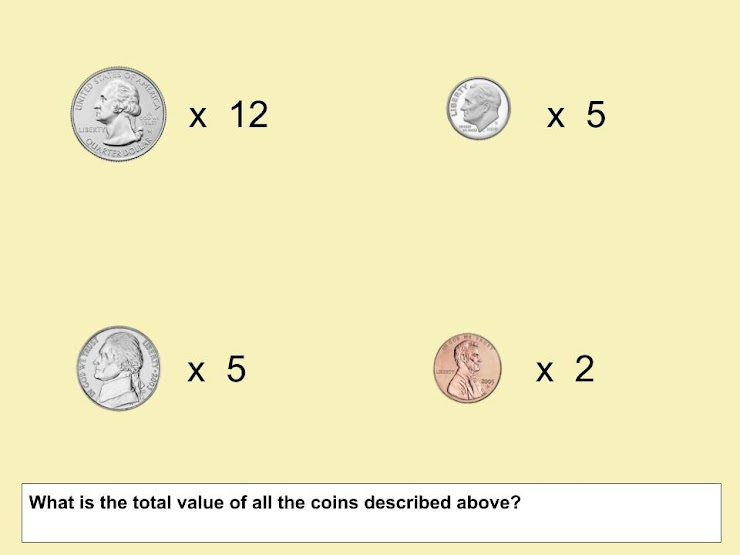7. In the space below, type the total value of the coins described below. (Be sure to use a dollar sign and decimal point in your answer.)
1 point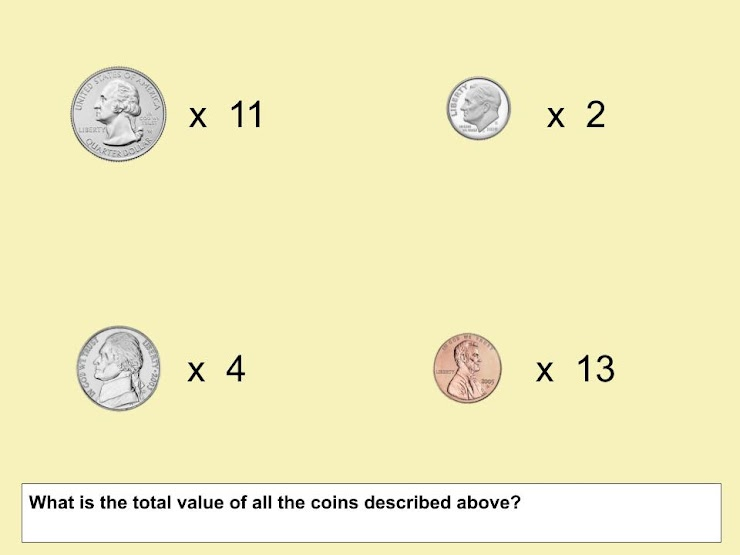8. In the space below, type the total value of the coins described below. (Be sure to use a dollar sign and decimal point in your answer.)
1 point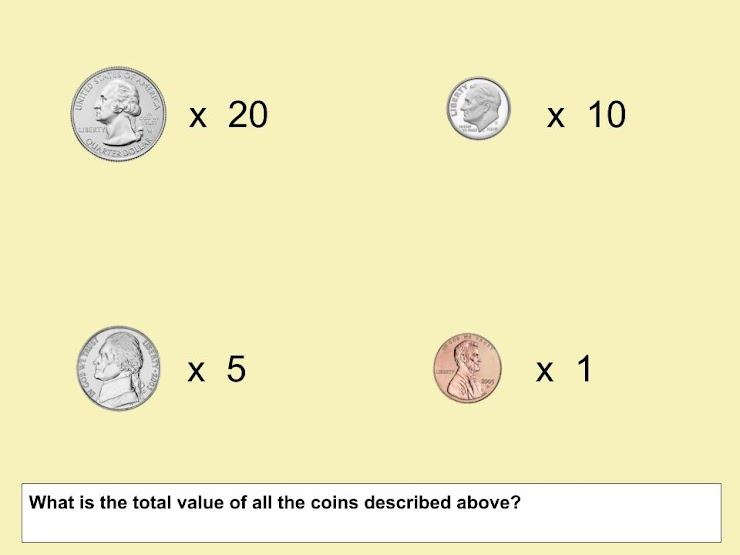9. In the space below, type the total number of quarters needed to answer this question, as a whole number.
1 point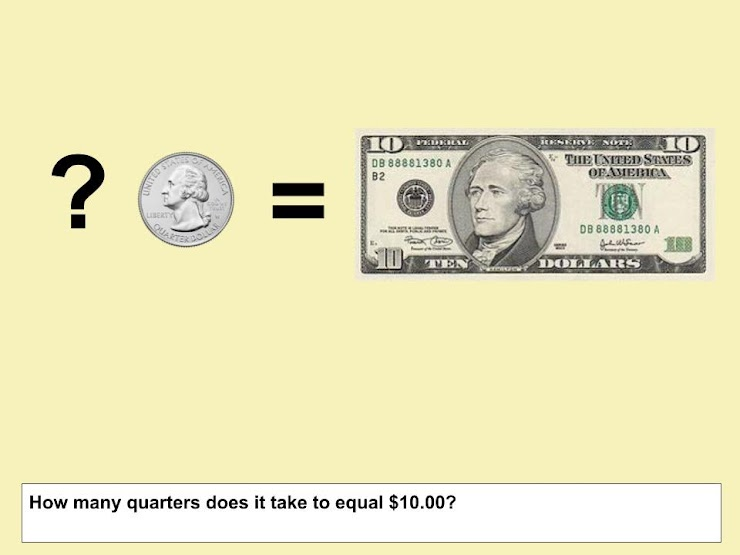10. What is the total value of the coins shown below?
1 point